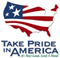# The National Flood-Frequency Program—Methods for Estimating Flood Magnitude and Frequency in Rural and Urban Areas in Georgia

#### U.S. Geological Survey Fact Sheet 169-98 August, 1999

This report is available in pdf 157KB.

# Introduction

Estimates of the magnitude and frequency of flood-peak discharges and flood hydrographs are used for a variety of purposes, such as for the design of bridges, culverts, and flood-control structures; and for the management and regulation of flood plains. To provide simple methods of estimating flood-peak discharges, the U.S. Geological Survey (USGS) has developed and published equations for every State, the Commonwealth of Puerto Rico, American Samoa, and a number of metropolitan areas in the United States. In 1993, the USGS, in cooperation with the Federal Emergency Management Agency and the Federal Highway Administration, compiled all current USGS statewide and metropolitan area equations into a computer program, titled “The National Flood-Frequency (NFF) Program” (Jennings and others, 1994).

Since 1993, new or updated equations have been developed by the USGS for various areas of the Nation. These new equations have been incorporated into an updated version of the NFF Program.

Fact sheets that describe application of the updated NFF Program to various areas of the Nation are available. This fact sheet describes the application of the updated NFF Program to streams that drain rural and urban areas in Georgia

## Rural Basins

Georgia is divided into four hydrologic regions (fig. 1) on the basis of previous flood-frequency studies, geology, soils, physiography, and analysis of flood-frequency regression residuals. Stamey and Hess (1993) developed regression equations for estimating peak discharges (QT), in cubic feet per second, that have recurrence intervals (T) that range from 2 to 500 years for ungaged, unregulated, non-tidal rural streams. The regression equations are not applicable to the Okefenokee Swamp in southeast Georgia. The regression equations also are not applicable to urban, channelized, or large main-stem streams or to streams where large sinkholes or depressions can affect the flood-frequency relation. These features can be identified by the study of geologic and topographic maps or by the reconnaissance of the basin.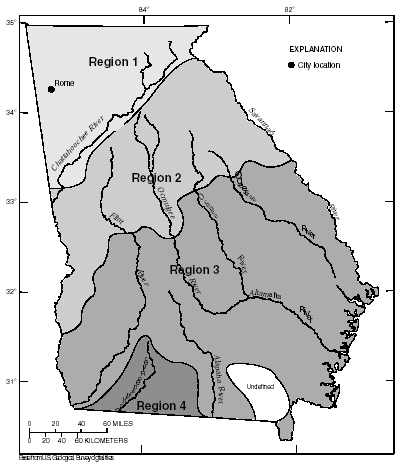Figure 1. Hydrologic regions for Georgia.

Drainage area (A) was found to be the most statistically significant explanatory watershed variable and is the only variable that is used in the regression equations. The drainage area is the total area that contributes runoff upstream of the stream site of interest. Drainage areas were measured in square miles for the equations determined by Stamey and Hess (1993), but the NFF Program will accept input and report results in either the inch-pound or metric system of units.

The regression equations, the average standard errors of prediction, the equivalent years of record, and the range of the drainage area that is applicable for use in the equations are shown in table 1. The average standard error of prediction is a measure of the average accuracy of the regression equations when estimating peak-discharge values for ungaged watersheds similar to those that were used to derive the regression equations. The equivalent years of record is the number of years of streamflow record needed to achieve the same accuracy as the regression equation. The standard error of prediction increases appreciably when drainage-area values that are outside the quoted range of data used to develop the equations are applied in the equation.

Regression equation Average standard error Equivalent years of record Applicablerange ofdrainage of prediction, in percent area, in square miles Table 1. Flood-peak discharge regression equations and associated statistics for streams that drain rural areas in Georgia (modified from Stamey and Hess, 1993) [QT, peak discharge for recurrence interval T, 2 to 500 years, in cubic feet per second; A, drainage area; Do., ditto] Q2 = 207A0.654 31 3 0.17–730 Q5 = 357A0.632 29 4 Do. Q10 = 482A0.619 29 5 Do. Q25 = 666A0.605 29 12 Do. Q50 = 827A0.595 30 14 Do. Q100 = 1,010A0.584 31 16 Do. Q200 = 1,220A0.575 33 17 Do. Q500 = 1,530A0.563 36 18 Do. Q2 = 182A0.622 33 4 0.10–3,000 Q5 = 311A0.616 28 7 Do. Q10 = 411A0.613 27 10 Do. Q25 = 552A0.610 28 14 Do. Q50 = 669A0.607 30 16 Do. Q100 = 794A0.605 33 17 Do. Q200 = 931A0.603 36 19 Do. Q500 = 1,130A0.601 40 21 Do. Q2 = 76A0.620 36 3 0.14–3,000 Q5 = 133A0.620 35 6 Do. Q10 = 176A0.621 35 10 Do. Q25 = 237A0.623 37 15 Do. Q50 = 287A0.625 38 18 Do. Q100 = 340A0.627 38 19 Do. Q200 = 396A0.629 40 19 Do. Q500 = 474A0.632 43 20 Do. Q2 = 142A0.591 25 8 0.25–2,000 Q5 = 288A0.589 19 27 Do. Q10 = 410A0.591 19 37 Do. Q25 = 591A0.595 21 43 Do. Q50 = 748A0.599 24 40 Do. Q100 = 926A0.602 28 37 Do. Q200 = 1,120A0.606 32 35 Do. Q500 = 1,420A0.611 37 32 Do.

The regression equations were developed by using peak-discharge data for streamflow-gaging stations with at least 10 years of record collected through September 1990 at 357 locations in Georgia and 69 locations in Alabama, Florida, North Carolina, South Carolina, and Tennessee.

Stamey and Hess (1993) developed weighting techniques to improve estimates of peak discharge at gaged locations by combining the estimates derived from analysis of gage records with estimates derived from the regression equations. The weights of these two independent estimates are based on the length of the gage record in years (N) and the equivalent years of records (EQ) of the applicable regression equation. The weighted estimate of peak discharge is computed as: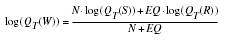where

 QT(W) is the weighted estimate for recurrence interval T at the gage location, QT(S) is the estimate of QT derived from analysis of the gage records, QT(R) is the estimate of QT derived from application of the regression equation, N is the number of years in the gage record, and EQ is the equivalent years of record (table 1).

The accuracy of the weighted discharge estimate, in equivalent years of record, is equal to N + EQ.

Stamey and Hess (1993) also showed how the weighted estimate for peak discharge at a gaged site can be used to improve estimates of peak discharge for an ungaged site on the same stream that has a drainage that is between 50 and 200 percent of the drainage area of the gaged site. The weighted estimate is computed as: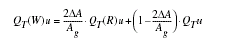where

 QT(W)u is the weighted estimate for recurrence interval T at the ungaged site, ΔA is the difference in drainage area between the gaged site (Ag) and the ungaged site, (Ag-Aungaged), QT(R)u is the peak flow estimate for recurrence interval T at the ungaged site derived from the applicable regional equation (table 1), QTu is the weighted estimate of peak discharge at the gaged site, QT(W), adjusted for the effect of the difference in drainage area between the gaged site and the ungaged site and computed as: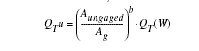where

b is the exponent of drainage area in the appropriate regression equation (table 1).

The NFF Program contains algorithms for each of the weighting computations. The equation estimates are used without adjustment where the drainage area at the ungaged site is not within one-half to two times the drainage area of the gaged site.

When the drainage area of the site of interest is in more than one region, a weighted estimate of the peak discharge should be computed. The equations for the appropriate regions should be applied independently using basinwide estimates of the required explanatory variables as if the entire basin was in each region. The weighted estimate is then computed by multiplying each regional estimate against the fraction of the drainage area in that region and summing the products. The NFF Program provides an algorithm for this computation.

Stamey and Hess (1993) summarized peak-discharge estimates derived from the peak-discharge records, the years of record, and the peak-discharge estimate derived from weighting the regression equation and station-record estimates for 357 sites located in Georgia. They also presented graphs of peak discharge as a function of drainage area for selected recurrence intervals for portions of the Ocmulgee, the Oconee, the Altamaha, and the Flint Rivers.

## Urban Basins

Georgia is divided into four urban hydrologic regions that correspond to the hydrologic regions developed by Stamey and Hess (1993). Inman (1995) developed regression equations for estimating peak discharges (QT), in cubic feet per second, that have recurrence intervals (T) that range from 2 to 500 years for the four hydrologic regions and a separate set of equations for the city of Rome, Ga. The equations are not applicable to basins with significant surface or embankment storage.

Drainage area (A), in square miles, is the total area that contributes runoff upstream of the location of the stream site of interest. In urban areas runoff from some subwatersheds may be diverted out of or into the drainage area of interest by stormwater sewers. The areas of such subwatersheds should be subtracted or added as appropriate to the drainage area of interest to compute the effective drainage area.

Total impervious area (TIA) is the percentage of the drainage area, A, that is covered by impervious surfaces and can be estimated by examination of maps or aerial photos and the use of the grid-sampling method or planimeters and field reconnaissance of the basin.

The regression equations and average standard errors of prediction are shown in table 2. The applicable range of basin variables used to develop the equations are shown in table 3. The regression equations were based on generalized least-squares (GLS) regression techniques used on synthetic peak-discharge data that resulted from calibrated rainfall-runoff model simulations using long-term rainfall and evaporation data for 65 sites in urban areas of Georgia. If the estimate of peak discharge using the rural equations (Stamey and Hess, 1993) exceeds the estimates derived from the application of the urban equations, use the rural peak-discharge estimates.

Regression equation Average standard error or prediction, in percent Table 2. Flood-peak discharge regression equations and associated statistics for streams draining urban basins in Georgia (modified from Inman, 1995) [QT, peak discharge for recurrence interval T, 2 to 500 years, in cubic feet per second; A, drainage area, in square miles; TIA, area that is impervious to infiltration of rainfall, in percent] Q2 = 167A0.73TIA0.31 34 Q5 = 301A0.71TIA0.26 31 Q10 = 405A0.70TIA0.21 31 Q25 = 527A0.70TIA0.20 29 Q50 = 643A0.69TIA0.18 28 Q100 = 762A0.69TIA0.17 28 Q200 = 892A0.68TIA0.16 28 Q500 = 1,063A0.68TIA0.14 28 Q2 = 107A0.73TIA0.31 40 Q5 = 183A0.71TIA0.26 36 Q10 = 249A0.70TIA0.21 35 Q25 = 316A0.70TIA0.20 33 Q50 = 379A0.69TIA0.18 33 Q100 = 440A0.69TIA0.17 33 Q200 = 505A0.68TIA0.16 34 Q500 = 589A0.68TIA0.14 34 Q2 = 145A0.70TIA0.31 35 Q5 = 258A0.69TIA0.26 31 Q10 = 351A0.70TIA0.21 31 Q25 = 452A0.70TIA0.20 29 Q50 = 548A0.70TIA0.18 29 Q100 = 644A0.70TIA0.17 29 Q200 = 747A0.70TIA0.16 28 Q500 = 888A0.70TIA0.14 28 Q2 = 54.6A0.69TIA0.31 34 Q5 = 99.7A0.69TIA0.26 31 Q10 = 164A0.71TIA0.21 32 Q25 = 226A0.71TIA0.20 30 Q50 = 288A0.72TIA0.18 30 Q100 = 355A0.72TIA0.17 30 Q200 = 428A0.72TIA0.16 30 Q500 = 531A0.72TIA0.14 30 Q2 = 110A0.66TIA0.31 34 Q5 = 237A0.66TIA0.26 31 Q10 = 350A0.68TIA0.21 30 Q25 = 478A0.69TIA0.20 29 Q50 = 596A0.70TIA0.18 28 Q100 = 717A0.70TIA0.17 28 Q200 = 843A0.70TIA0.16 28 Q500 = 1,017A0.71TIA0.14 28

 Hydrologic study region Drainage area, in square miles Table3. Range of explanatory variable for which regression equations are applicable [do., Do., ditto] Region 1 0.04–19.1 1.00–62 Rome do. Do. Region 2 do. Do. Region 3 do. Do. Region 4 do. Do.

Inman (1995) presented rainfall-runoff model parameters, explanatory watershed variables, and peak-discharge estimates (QT) for the 65 streamflow-gaging stations used to develop the equations, and analyses of the sensitivity of the equation to errors in estimates of watershed variables.

## References

Inman, E.J., 1995, Flood-frequency relations for urban streams in Georgia–1994 update: U.S. Geological Survey Water-Resources Investigations Report 95-4017, 27 p.

Jennings, M.E., Thomas, W.O., Jr., and Riggs, H.C., comps., 1994, Nationwide summary of U.S. Geological Survey regional regression equations for estimating magnitude and frequency of floods for ungaged sites, 1993: U.S. Geological Survey Water-Resources Investigations Report 94–4002, 196 p.

Stamey, T.C., and Hess, G.W., 1993, Techniques for estimating magnitude and frequency of floods in rural basins of Georgia: U.S. Geological Survey Water-Resources Investigations Report 93-4016, 75 p.

U.S. Geological Survey

Office of Surface Water

415 National Center

Reston, Virginia 20192

(703) 648-5301

USGS hydrologic analysis software is available for electronic retrieval through the World Wide Web (WWW) at: http://water.usgs.gov/software/ and through anonymous File Transfer Protocol (FTP) from water.usgs.gov (directory: /pub/software). The WWW page and FTP directory from which the National Flood-Frequency software and user documentation can be retrieved are http://water.usgs.gov/software/nff.html and /pub/software/surface_water/nff, respectively.

Additional earth science information is available from the USGS through the WWW at http://www.usgs.gov/ anonymous or by calling 1-888-ASK-USGS.

 AccessibilityFOIAPrivacyPolicies and Notices U.S. Department of the Interior, U.S. Geological Survey Persistent URL: Page Contact Information: Contact USGS Last modified: Tuesday, November 29 2016, 05:53:57 PM